Smartick is a fun way to learn math!Jul08

# Dividing with Decimals Using Money as an Example

In today’s post, you are going to learn how to solve division problems with decimals in the dividend, in other words, dividing a decimal number by a whole number.

In order for you to understand the process well, I am going to show you how we explain dividing with decimals in the interactive Smartick tutorial which uses money as a visual aid.

You will learn how to solve an easy example so you can understand it better by seeing each step in detail.

If you want to review the different types of division with decimals, you can check out this post.

### Dividing with decimals in the dividend using money as a visual aid

In the interactive tutorial, we learn that Otto has gone to get ice cream with Eva and Amy.They have bought 3 ice creams at the same price and in total, they cost $3.72. So now they want to know how much each ice cream cost. Therefore, to find out, they need to divide 3.72 by 3. To represent the division process step by step, they display the coins that make up$3.72 grouped by the type of coin.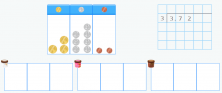And they’re going to distribute the coins into 3 equal groups.

#### Let’s start with the $1 coins!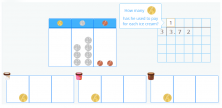Since 1 coin corresponds to each group, it means that they have each spent one$1 coin on ice cream, with none left over.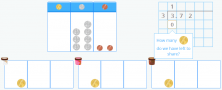#### Now let’s distribute the dimes!

2 coins correspond to each group with 1 left over.

Since they are dimes and each one is equivalent to $0.10, to represent them as a quotient, put a period after the 1 (two dimes is the same as$0.20).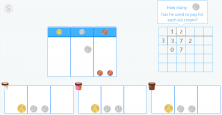So they’ve each spent two dimes on ice cream, with 1 left over.In order to distribute the 1 dime that is left between the 3 groups, they change it to 10 pennies. Now there are 12 pennies to distribute.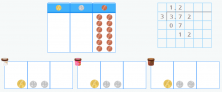#### Let’s distribute the pennies!

They distribute these 12 pennies into the 3 groups with each group receiving 4 coins.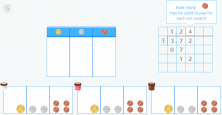So they have each spent 4 pennies on ice cream with nothing left over.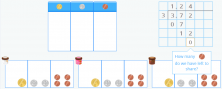In total, according to the division problem, they have each spent \$1.24 on ice cream.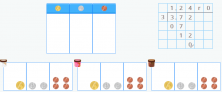And that is our example of dividing with decimals, I hope you found it easy!

If you want to continue enjoying mathematics with interactive tutorials and exercises, login to Smartick and try it for free.